LIBRARY

# External Ramp Autotuning for Current Mode Control of Switching Converters

Year: 2013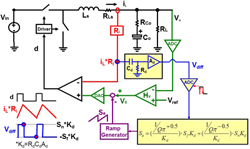Fig. 1. Peak Current Mode Control with Digital Autotuning Block.
Many current mode controllers try to cover a wide input and output voltage operation range. However, for peak current-mode control when the duty cycle (D) is above 0.5, subharmonic oscillations occur and the quality factor (Q2) of the high-frequency double pole in the control to output voltage transfer function (Vo(s)/Vc(s)) becomes negative as shown in (1). An external ramp slope (Se) is added to help stabilize the system. Conventionally, to ensure the stability for a wide duty cycle range, a fixed Se over-compensates the double pole, so the system bandwidth (BW) is limited. Therefore, several adaptive ramp strategies have tried to make Se tracks Sf change, so proper Se can be chosen for different D. Figure (1) shows that when Se=Sf, Q=2/π. However, the tracking error from the inductor variation still causes overcompensation, because Q is no longer constant when Se Sf.

This paper discovers that as long as Se is tuned with the real current slope change (Sn, Sf), Q2 can be well-controlled, since input voltage, output voltage, and inductance variations are exhibited in the current feedback signal (iL*Ri). The high frequency characteristic of Vo(s)/Vc(s) is fixed and immune to those variations, so a high BW design can be achieved. Sn and Sf are sensed by differentiating the current feedback signal with the RC circuit and then sample-and-holding the two slope values. After that, the external ramp for desired Q2 can be calculated by the simple control law in Figure 2.

Finally, simple digital and analog implementations are proposed to realize the autotuning method into peak current mode, valley current mode, and average current mode controllers. Also, they can be used in different topologies without changing control law and tuning circuitry. The simulation and experimental results verified the effectiveness of proposed method, as shown in Fig. 3.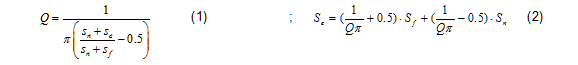Where Sn and Sf are the rising and falling slope of the inductor current respectively. For the buck converter, Sn=(Vin-Vo)Ri/L and Sf=VoRi/L. Ri is the current sensing gain and L is the filter inductance.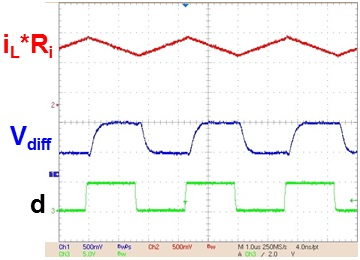Fig. 2. Measurement of differentiated waveform (Vdiff).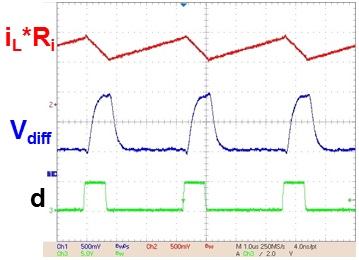Fig. 2. Measurement of differentiated waveform (Vdiff).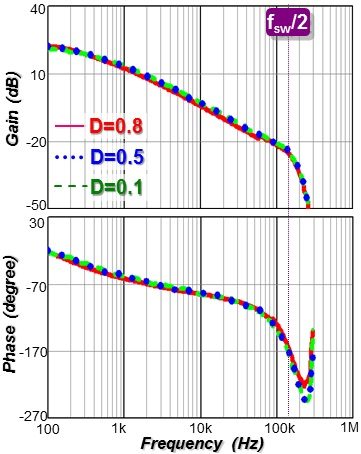Fig. 3. Measured Vo(s)/Vc(s) with digital autotuning block.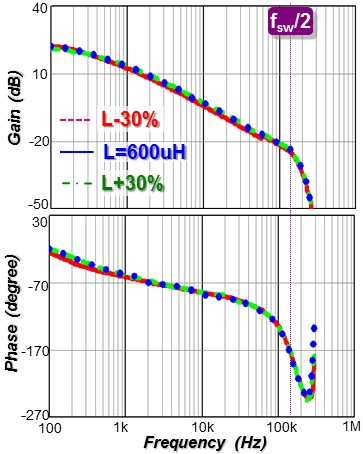Fig. 3. Measured Vo(s)/Vc(s) with digital autotuning block.